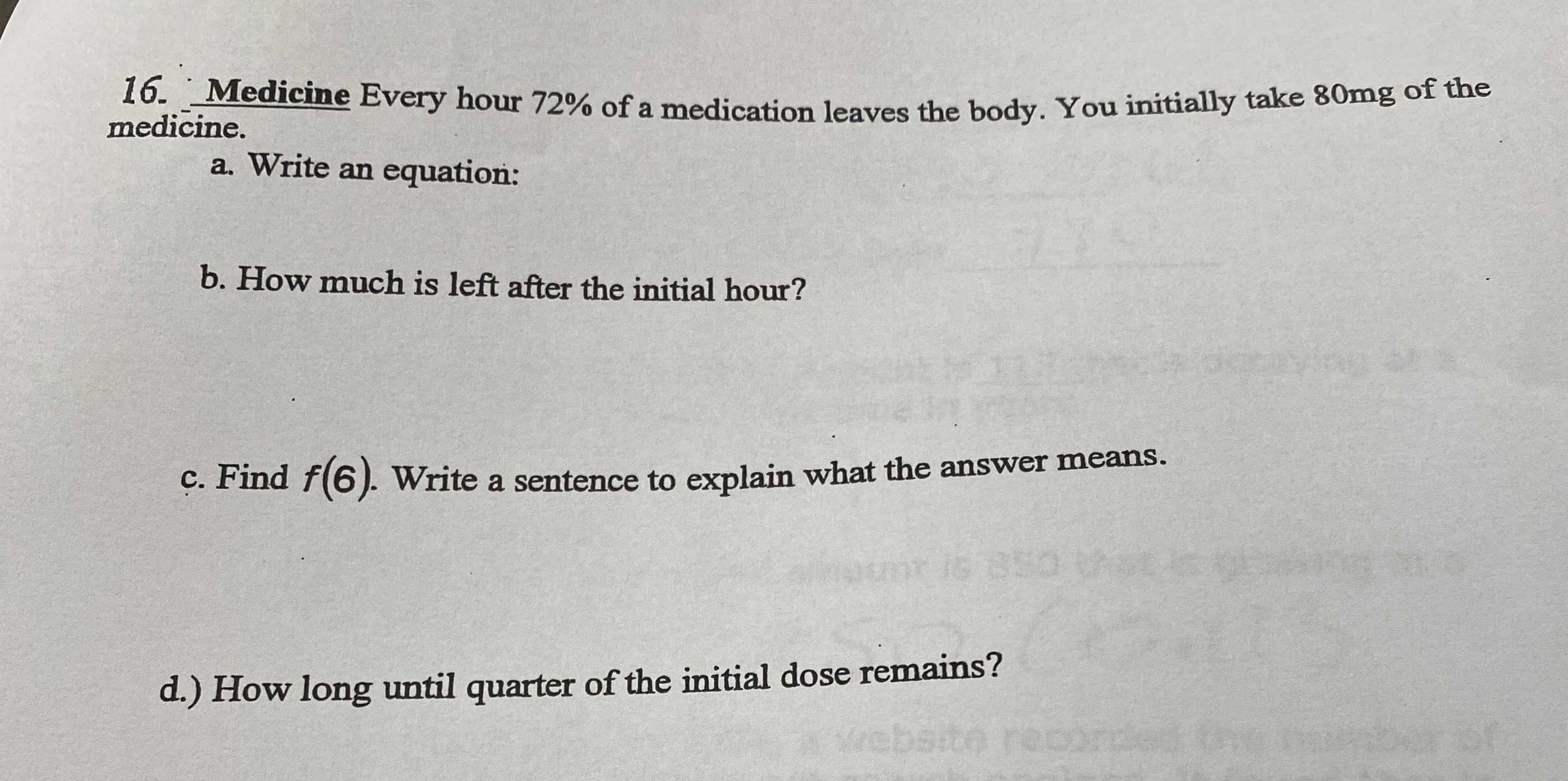### ¿Todavía tienes preguntas de matemáticas?

Pregunte a nuestros tutores expertos
Algebra
Pregunta16. Medicine Every hour $$72 \%$$ of a medication leaves the body. You initially take $$80 mg$$ of the medicine.

a.Write an equation:

b. How much is left after the initial hour?

c. Find $$f ( 6 )$$ . Write a sentence to explain what the answer means.

d.) How long until quarter of the initial dose remains?

a.

if the number of hours is h,

$$y = 80(0.72)^h$$

b.

After one hour = 80(0.72) = 57.6 mg

c.

11.15 mg.  The amount of the medication left after 6 hours is 11.15 mg

d.

After 4.22 hours

Solución
View full explanation on CameraMath App.